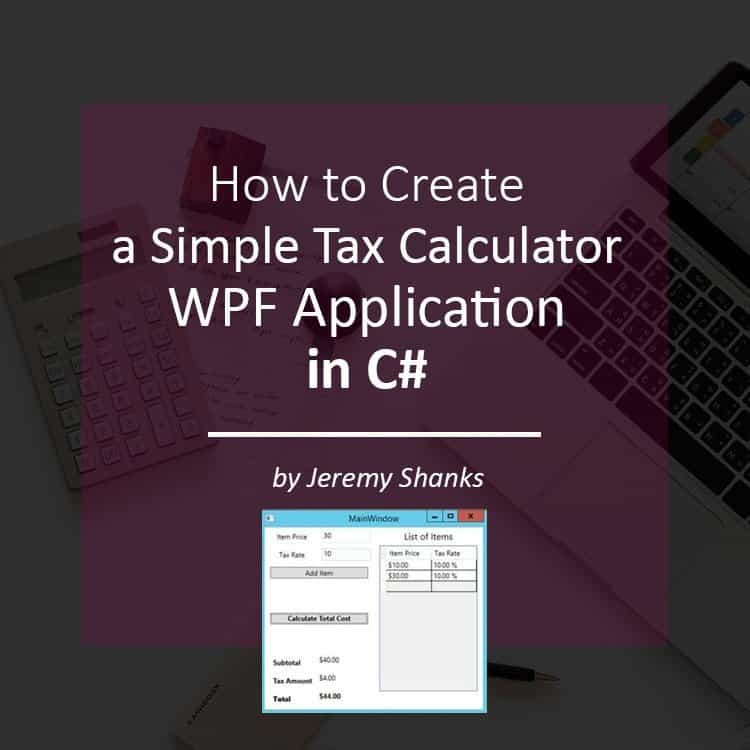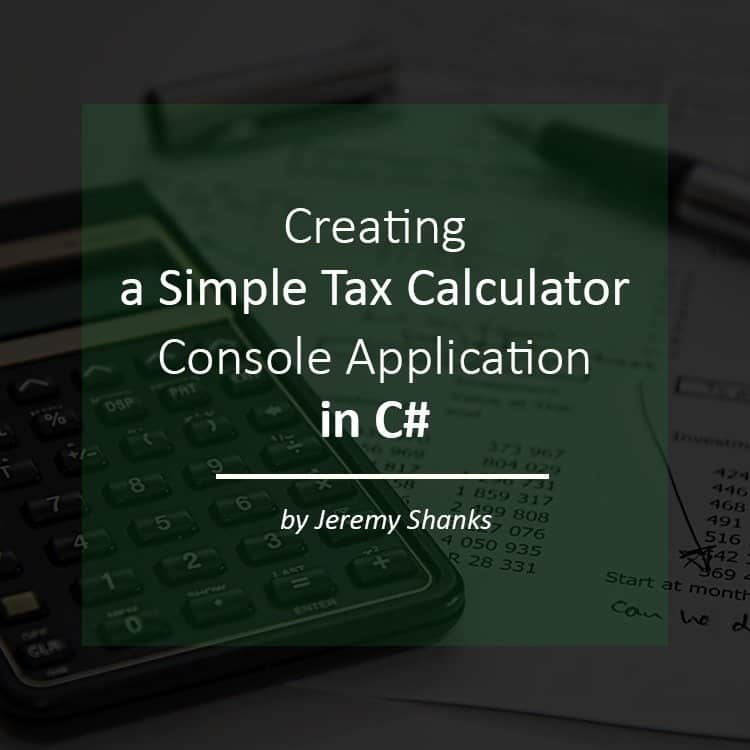How to Create a Simple Tax Calculator WPF Application in C#In my last tutorial, I showed you how to create a simple tax calculator console application in C#. As promised, in this tutorial I will show you how to make that same tax calculator with a Graphical User Interface (GUI) and with a few feature enhancements. By the end of this tutorial, you will be able to run your tax calculator and calculate the grand total of a list of items with different tax rates for each item.Continue reading

Creating a Simple Tax Calculator Console Application in C#In this tutorial, I will show you how to create a simple tax calculator in C# using Visual Studio. I will show you how to get input from a user and do some calculations on that input. By the end of this tutorial, you will be able to run your tax calculator to calculate the grand total of an item given a specific tax rate.Continue reading